Scan QR code or get instant email to install app

Question:

# Which has more volume: a 10-inch tall can with a diameter of 10 inches, or a 7\u00D710\u00D710-inch box?

A can
explanation

1212The comparison is made between two solids, one a cylinder (the can) and the other a rectangular box. To help us visualize the comparison, let's use these sketches:The formula for the volume of a cylinder is: Volume = \uD835\uDF0B \u00D7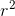\u00D7 H, where H is the height of the cylinder The problem does not provide the radius. Instead, it gives the diameter as 10. We know that the radius of a circle is 1/2 of its diameter, or: r =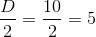inches We go back to the formula for volume and plug in the values r = 5 and \uD835\uDF0B = 3.14: Volume = 3.14 \u00D7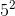\u00D7 10 = 785 cubic inches Notes: We may actually directly solve for the volume using the formula V =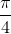\u00D7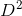\u00D7 H and get the same answer. Now, we're ready to solve for the volume of the box: Volume = length \u00D7 width \u00D7 height = 10 \u00D7 10 \u00D7 7 = 700 cubic inches Now, we see that the volume of the can is greater than that of the box.